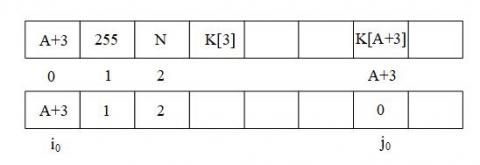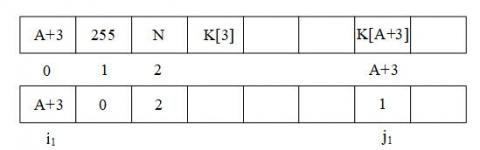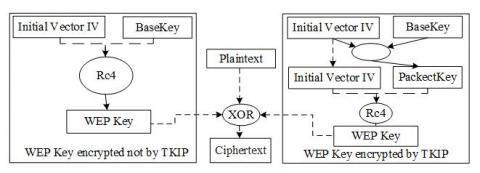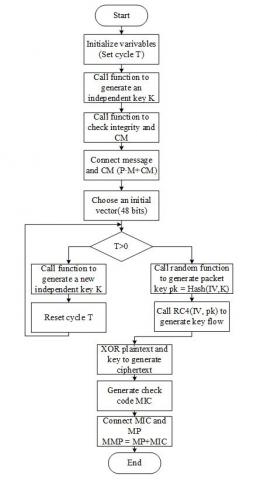# Analysis and Improvement of Wired Equivalent Privacy Protocol

Analysis and Improvement of Wired Equivalent Privacy Protocol
School of Computer Science and Technology, Harbin University of Science and Technology, Harbin 150080, China

Corresponding Author Email:
luozhiyongemail@sina.com
Page:
11-14
|
DOI:
https://doi.org/10.18280/rces.060103
19 December 2019
|
Accepted:
25 February 2019
|
Published:
31 March 2019
| Citation

OPEN ACCESS

Abstract:

The aim of this study was to investigate the mechanism for the security of a wireless network. The research of the wired equivalent privacy algorithm and statistical probability model approach was adopted, in addition, a new WEP encryption algorithm based on this was introduced. The results revealed that this encryption algorithm is insufficient, and concluded the time to crack the key and the amount of encryption package needed capturing. The findings of this study may make wireless network more safety in the aspect of data transmission, thus it may be beneficial to widespread of the wireless network.

Keywords:

wireless network, WEP protocol, RC4 algorithm, statistical probability model

1. Introduction

Recently, Wireless LAN based on the IEEE 802.11 standard is widely used. Due to its fast transmission speed and long transmission distance, it adopted more than any others (such as Bluetooth and infrared ray). However, its security is a big problem people care about. Hackers can enter WLAN through special channels and illegal steal user personal information. A better-encrypted method can be beneficial to the wide application of the wireless network. Thus, to ensure the security of WLAN is the focus of current work.

Compared with WPA2, WEP has not much processing power and only applies a static key [1-2]. In paper , the literature analysis the WEP mechanism and proposes its security vulnerabilities, it also points out that the algorithm has no protection against replay attacks. In order to eliminate the security threats, some work has been done. In paper , a new approach which is adding an additional layer over RC4 that is core part in WEP, it shows using this methodology, the security would be enhance greatly compared with the original algorithm. In the situation of using WEP in e-learning courses, some researchers found that its better when choosing the 24-bits initialization vector .

In paper , a concept of improved strong IVs has been proposed, it can avoid the reduction of throughput. In WEP, the SHA1 algorithm is more suitable than CRC-32, it has lower end-to-end delay and high packet delivery ratio . When the size of key increases, using the SHA3 algorithm is better than SHA1 and CRC-32 in security and performance . In paper , a new implementation of RC4 is proposed, the main approach is to add its randomness, this lead to improved output than standard WEP. When change the key to 64-bits dynamic key consist of initial vector and fixed key, it shows that this method can protect RC4 from brute force .

In order to improve the security problem of wireless network, a new WEP encrypted method is proposed, called TKIP. In our approach, we change the initial vector to 48 bits, generate a new key for every client and use a check code to maintain the integrity of the message. Our main contributions can be summarized as follows: (1) We analysis the whole process of the WEP protocol and draw a conclusion that the method cracked the key cannot always recovery it. (2) We utilize a statistical probability model to reduce a large amount of calculation problem. (3) We list the recovery algorithm of WEP key and point that the cracking time is almost the same no matter how many bits it is. (4) We introduce three improvements of the TKIP algorithms.

The remainder of this paper is organized as follows: In Section 2, an analysis of the WEP protocol as well as the way it encrypts, cracks and recoveries the key are detailed. Section 3 introduces the statistical probability model; analyzes from the perspective of probability how many samples it needs to crack the key. In Sect.4, an implementation of the WEP key recovery algorithm is listed as well as the results of experiments. Section 5 shows three improvements in the improved algorithm and gives its flow chart. Last, we conclude this paper with a discussion in Section 6.

2. Wep Protocol Analysis

WEP protocol uses the RC4 algorithm to encrypt data and uses CRC-32 algorithm to verify the integrity of data. RC4’s state space is too large so that it will have log2 (28!×(28)2)≈1700 states if its substitution-box with 8 bits. Mass software adopts this algorithm because of it's convenient and fast.

There is one key K used in WEP encryption, it shared by all mobile sites and AP, that is to say, they must all know this K. In order to get an encrypted frame, we need to calculate the checksum C(M) of a plaintext M firstly, then append C(M) to plaintext M, denoted by M·C(M). Afterwards, network adapter chooses an initial vector IV to add to the front of key K, called “Packet key”, RC4 algorithm uses it to initialize substitution-box and generates an output sequence of random numbers. This sequence xor checked plaintext to generate the ciphertext:

C=[M·C(M)]⊕RC4(IV·K)

Actually, the WEP data transported is consisting of IV and ciphertext (IV added before the C and transported in the form of plaintext).

In the currently used WEP protocol, bag key is an RC4 key that usually consists of 24 bits IV and 40 (or 104) bits user key. If only care about the first byte of the encrypted data, meanwhile its corresponding plaintext can obtain easily, we will get the first byte of the random sequence produced by PRGA algorithm in RC4, it called OUTPUT. This OUTPUT only relies on three particular elements S(1), S(1), S{S(1)+S[S(1)]} in substitution-box, Figure 1 shows that the state of substitution-box after KSA algorithm.

## 1.jpgFigure 1. The state of substitution-box

The Z in Figure 1 is obviously OUTPUT. Generally speaking, its output is totally random. However, some special IV will leak the key’s WEP package and analysis it to get key. Now considering such a situation, we can know OUTPUT after I turns in the KSA algorithm. Of course, the probability of this “unchanged state” is very small, but in some special IV cases, its probability will be 5 %, of course ,the remain 95 % probability is shown in Figure 1, three numbers X, Y, Z will continue joining exchange, to make the first output of random sequence truly stochastic.

Supposing we know IV with i bytes and user’s key K(0), K(1),…, K(j-1)(the unit is byte). Now we want to obtain the B key K(B), the method of cracking is finding IV, after i turns, we have:

S(1),<I (1)

S(1),+SI[S(1),]=I+B (2)

We can obtain an “unchanged state” after I+B turns in a case of high probability, then the first output of RC4 probably be:

Out=SI+B-1(jI+B)=SI+B-1[jI+B-1+K(B)+SI+B-1(I+B)]

If know Out(namely OUTPUT is known), jI+B-1and SI+B-1, we can get:

K(B)=S-1I+B-1(Out)-jI+B-1-SI+B-1(I+B) (*)

S-1I+B-1 denotes the position that Out appeared within the replacement SI+B-1. The correct rate of this results will be 5 % approximately, we can obtain correct K(B) by capturing and analyzing enough WEP package of different IV.

Now considering 64 bits WEP particularly (3 bytes plaintext IV), the key recovery method is as follows:

Supposing we know the first A bytes [K(3),…, K(A+2), A=0 at first] of user key with 5 bytes, in order to obtain the A+1 key K(A+3), we analysis the IV which the form is (A+3, 0xFF, N), where N ∈0x00…0xFF, namely N is at will. Now observing the first A+3 rounds of KSA algorithm.

In the first round, j adds A+3, then S(i) and S(j) exchanged. At this stage, substitution-box is shown in Figure 2, where the first row represents IV and key supplied to KSA algorithm, the middle row represents No., the next row represents the state of substitution-box, and in the bottom row, two subscripts represent the current position of i and j.

## 2.jpgFigure 2. The new state of substitution-box

After that enter the second round, adding 1 to i, now the increment of j is 0, so we can obtain the structure shown in Figure 3 when finish exchanging S(i) and S(j).

## 3.jpgFigure 3. The state of substitution-box after exchanging

Due to the value of N and K(3),…,K(A+2) have been known, we can calculate the state SA+2 and jA+2 of substitution-box after A+2 rounds. At the round of A+3, the increment of j is SA+2(iA+3)+K(A+3), then exchange S(i) and S(j), we can get the structure shown in Figure 4:

## 4.jpgFigure 4. The structure of substitution-box

After A+3 rounds, condition (1) and (2) will be met, so we can reach the “unchanged state”, then the K[A+3] can be calculated by the formula (*). Different N makes different j, so that we can recovery K(A+3) through different N.

This crack method is cracking byte by byte, that is to say, the crack of latter byte must rely on the keys of all bytes we already have known.

3. Statistical Probability Model

The previous section points out that the way to crack the key not always recovery the key. Thus, for that IV which has the form of (A+3, 0xFF, N), we only have a 5 % probability to calculate the correct result. In other words, we can always calculate one value between 0 and 255 (totally 256) according to formula(*), but guarantee that it is correct under 5 % probability. The value is denoted by δ, namely the probability of δ’s appearance is 5 %. And the probability of the incorrect is 95 %, in this situation, the value is one of other 255 numbers excepts δ, and all the number appears in the same probability which is 0.95/255 *100 % ≈0.37255 %. The probability of δ’s appearance is greater than the others apparently.

In order to crack the K(A+3), we need a IV in the form of (A+3, 0xFF, N), where N∈0x00…0xFF. We call this IV a sample, the different sample represents different value IV.

As a result of that, for which n, whether we can both guarantee that the counts of the value are the most and it appears in a max probability, that is apparently a probability question.

Supposing we have n experiments, the times δ appearing in those n experiments denoted by Xδ. Xi is the times I appeared in this n experiments, i=0,…,255, i≠δ. It can clearly be seen that Xδ and Xi’s possible values are 0,1,…,n. Obviously, p(Xδ=k)=(nk)pk1(1-p1)n-k, k=0,1,2,…,n. P(Xi=k)=(nk)pk2(1-p2)n-k, k=0,1,2,…,n, Xδ∽b(n,p1), Xi∽b(n,p2), i=0,…,255, i≠δ namely. Now we want to calculate P(Xδ＞max(Xi)).

Because Xδ and Xi are independent, in order to calculate the formula listed upper, we must calculate the joint probability density of 256 random variables first. The calculation is too complicated, almost unable to complete.

However, we can fix the issue using the central limit theorem here. According to the De Moivre-Laplace theorem, if random variable ηn obey binomial distribution with parameters n and p, then $\frac{{{\eta }_{n}}-np}{np(1-p)}$ obey normal distribution approximately when n is big enough. Because p1 and p2 are both little here, so we can believe that when n≥10, $\frac{{{X}_{\delta }}-n{{p}_{1}}}{\sqrt{n{{p}_{1}}(1-{{p}_{1}})}}$ and $\frac{{{x}_{i}}-n{{p}_{2}}}{\sqrt{n{{p}_{2}}(1-{{p}_{2}})}}$ obey N(0,1) normal distribution. Therefore:

$p(\frac{{{x}_{\delta }}-n{{p}_{1}}}{\sqrt{n{{p}_{1}}(1-{{p}_{1}})}}>\alpha )=\Phi (\alpha )$

$p(\frac{{{x}_{i}}-n{{p}_{2}}}{\sqrt{n{{p}_{2}}(1-{{p}_{2}})}}>\alpha )=\Phi (\alpha )$

If $n{{p}_{1}}-\alpha \sqrt{n{{p}_{1}}(1-{{p}_{1}})}>n{{p}_{2}}-\alpha \sqrt{n{{p}_{2}}(1-{{p}_{2}})}$ then Xδ> Xi at the possibility $\Phi (\alpha )$ for all. i≠δ

So the solution is $n>{{\left( \alpha (\frac{\sqrt{{{p}_{1}}(1-{{p}_{1}})}+\sqrt{{{p}_{2}}(1-{{p}_{2}})}}{{{p}_{_{1}}}-{{p}_{2}}}) \right)}^{2}}\approx 36{{\alpha }^{2}}$

When n = 20, the possibility that we can crack the key is 75 %, when n =36, the possibility is 84 %, and when n=144, the possibility is 97.7 %. Thus, in order to crack a key with one byte, we must need 20 samples at least, 36 samples are recommended. If we have 144 samples, the key can be certainly cracked.

4. Wep Key Recovery Algorithm

As mentioned in the first section, we can easily obtain the plaintext corresponding to the first byte of the WEP package. According to the experiments, the plaintext corresponding to the encrypted WEP package always has an 802.2 header, also called SNAP header. For IP network, the header consists of 8 bytes as follows: 0xAAAA, 0x0300, 0x0000, 0x0800. The plaintext corresponding to the first byte of WEP package is 0xAA always. Then we can obtain the first byte of the random sequence generated by PRGA through xor 0xAA with the first byte of WEP package. It’s the OUTPUT mentioned before. If it is IPX network, the first byte of SNAP header should be 0xFF or 0xE0.

The implementation algorithm is as follows:

RecoverWEPKey()

Key(0…KeySize)=0

for KeyByte = 0…KeySize

Counts (0…255)=0

for eachpacket→P

if P.IV∈[(KeyByte+3, 0xFF, N)  N∈0x00…0xFF]

Counts[Resolved(P,Key)]+=1

Key(KeyByte)=IndexOfMaximumElement(Counts)

Return Key

Verify(Key)

The Resolved function here is the calculation process mentioned in the first section, its return value is the result calculated by the formula(*). The Verify function checks whether the key calculated is correct, we can use the method of FCS, or use this key cracked WEP package to observe whether the header of SNAP and IP is correct.

As a result of experiments, if it has 30 samples for every byte (tips: repeat sample only count once), the key can be cracked basically, the number of captured WEP package is about 4 million, and no matter 40 or 104 bits’user key, the crack time is no different, both in 1 second.

5. Wep Key Recovery Algorithm

Because of the shortage WEP algorithm inhered, we need to improve it in the practical applications.

5.1 Improved algorithm

We use a protocol called “Temporal Key Integrity Protocol”, it strengthens the security of the WEP key. It changes the approach getting the key and keeps changing the key, and for preventing the forgery of the package, it strengthens the whole check function of the message, this makes the security of wireless network increasing substantially. Figure 5 is the comparison diagram of WEP and TKIP to encrypt the data.

Now let us see what changed in the TKIP compared with WEP.

(1) 48 bits initial vector

In TKIP, we increase the 24 bits’ initial vector to 48 bits, which makes the probability of collision reduced and increases security greatly.

(2) Generation and distribution of each package

TKIP generates a new independent key for each client periodically, and uses it to encrypt each frame of 802.11, this can avoid the situation that not change the key when using WEP for several weeks or months.

(3) Integrity code of message

Using MIC to prevent hackers, we add a 4 bits check code in frame body, the sender confirms MIC according to frame body, and plugs this MIC in frame body. Receiver confirms whether accept this frame according to MIC, it first checks whether message match MIC, then conducts information integrity check. If matched, data is completely transmitted and it can be accepted normally.

## 5.jpgFigure 5. A schematic comparison of WEP and TKIP

5.2 Flow chart of improved WEP algorithm

Figure 6 is the flow chart of the WEP algorithm applicated in practical, we will not introduce it anymore.

6. Conclusion

We analysis the shortage of WEP according to the practical, and draw a conclusion by a statistical model that it can always crack the key if intercept 36 samples. Moreover, we design an improved WEP algorithm based on it, it shows that the wireless network can more safety transmit using this algorithm.

## 6.jpgFigure 6. Flow chart of improved WEP algorithm

Acknowledgment

This work was supported in part by the Heilongjiang Province Foundation for Returnees (No.LC2018030), the National Natural Science Foundation of China (No.61772160).

References

 Gali TAB, Babiker AA, Mustafa N. (2015). A comparative study between WEP, WPA and WPA2 security algorithms. International Journal of Science and Research ISSN 2319-7064.

 Luo ZY, You B, Liu JH. (2016). Research of the Intrusion Tolerance State Transition System Based on Semi-Markov. Transactions of Beijing Institute of Technology 36(07): 712-717. https://doi.org/10.15918/j.tbit1001-0645.2016.07.010

 Sari A, Karay M. (2015). Comparative analysis of wireless security protocols: WEP vs WPA. International Journal of Communications, Network and System Sciences 8(12): 483. https://doi.org/10.4236/ijcns.2015.812043

 Garg A. (2016). A novel approach to secure WEP by introducing an additional layer over RC4. 2016 3rd International Conference on Computing for Sustainable Global Development (INDIACom). IEEE 551-555.

 Yamada M, Oda T, Liu Y, et al. (2016). Performance evaluation of an IoT-based e-Learning testbed considering OLSR and WEP Protocols. 2016 10th International Conference on Innovative Mobile and Internet Services in Ubiquitous Computing (IMIS). IEEE  335-341. https://doi.org/10.1109/NBiS.2016.22

 Watanabe Y, Iriyama T, Morii M. (2017). Proposal of WEP Operation with Strong IV and Its Implementation. Journal of Information Processing 25: 288-295. https://doi.org/10.2197/ipsjjip.25.288

 Grover A, Singh S. (2015). Comparative analysis of CRC-32 and SHA-1 algorithms in WEP. Advanced Engineering Technology and Application (1): 1-6.

 Kumar A, Arora V. (2015). Analyzing the performance and security by using SHA3 in WEP. IEEE International Conference on Engineering and Technology (ICETECH). IEEE 1-4. https://doi.org/10.1109/ICETECH.2015.7275026

 Hashem SH. (2017). A proposed modification on RC4 algorithm by increasing its randomness. Al-Rafidain University College for Sciences (39): 349-372.

 Garg A. (2015). A novel approach to enhance security in WEP. International Conference on Green Computing and Internet of Things (ICGCIoT). IEEE 585-588. https://doi.org/10.1109/ICGCIoT.2015.7380532